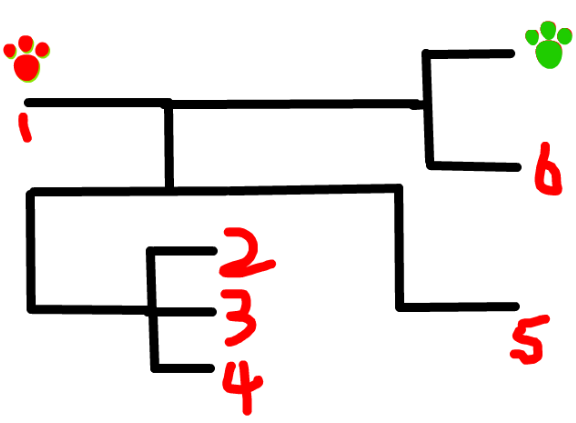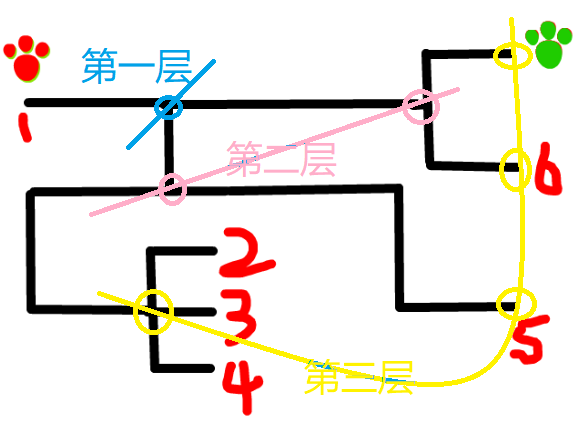# C语言-广度优先搜索(BFS)

0x00、仍然从迷宫说起0x01、广度优先搜索的实现方式0x02、使用C语言实现广度优先搜索

``````void BFS(int s){
queue<int> q;
q.push(s);
while(!q.empty()){
取队首;
访问队首;
弹出队首;
队首元素下一层级的元素全部入队;
}
}``````

0x03、举个例子

0 1 1 1 0 0 1        左边的6*7矩阵中

0 0 1 0 0 0 0        "块"的个数为4

0 0 0 0 1 0 0

0 0 0 1 1 1 0

1 1 1 0 1 0 0

1 1 1 1 0 0 0

``````int X[] = {0, 0, 1, -1};
int Y[] = {1, -1, 0, 0};``````

``````for(int i = 0; i < 4; i++){
newX = nowX + X[i];
newY = nowY + Y[i];
}``````

``````int n, m;    //矩阵大小为n*m
typedef struct{
int data;
bool flag;
} point;

point matrix;``````

``````typedef struct{
int x, y;
} node;
node Node;``````

``````void BFS(int x, int y){
queue<node> Q;    //定义队列
Node.x = x, Node.y = y;    //当前点的坐标
Q.push(Node);    //将该点压入队列
matrix[x][y].flag = true;    //将该点标记设置为 已入队
while(!Q.empty()){
node top = Q.front();    //取出队首
Q.pop();                //队首出队
for(int i = 0; i < 4; i++){    //循环4次，遍历四个方向
int newX = top.x + X[i];
int newY = top.y + Y[i];    //newX和newY在这四次循环中分别是4个方向上的点
if(judge(newX, newY)){    //如果这个点需要访问
Node.x = newX, Node.y = newY;    //让下一步处理的点变成这个需要等待处理的点
Q.push(Node);    //将这个点压入队列，在队尾等待处理。
matrix[newX][newY].flag = true;    //标记这个点已经入队。以后无需判断了
}
}
}
}``````

1，已入队的、曾经判断过的（flag=true）无需再次判断

2，该点为0的，肯定不是一个块的某个元素，无需判断

3，超过矩阵边界，会造成数组溢出，直接返回false，不可以判断

``````bool judge(int x, int y){
/*数组越界情况*/
if(x >= n || x < 0 || y >= m || y < 0)
return false;
/*当前位置为0，一定不是块元素，无需判断*/
if(!matrix[x][y].data)
return false;
/*当前位置已经判断过了，或者已入队，无需重复判断*/
if(matrix[x][y].flag)
return false;
/*以上都不满足，说明是一个从未判断过，并且数值为1的点，返回真*/
return true;
}``````

OK，上面我们完成了广度优先搜索算法的全部步骤。下面我们来完成主函数：

``````int main(){
cin >> n >> m;    //输入矩阵大小
for(int x = 0; x < n; x++)
for(int y = 0; y < m; y++){
cin >> matrix[x][y].data;
matrix[x][y].flag = false;
}
int result = 0;    //块数
for(int x = 0; x < n; x++){
for(int y = 0; y < m; y++){
if(matrix[x][y].flag == false && matrix[x][y].data == 1){
result++;
BFS(x, y);
}
}
}
cout << "块数为：" << result << endl;
return 0;
}``````

``````#include <iostream>
#include <queue>
using namespace std;

/*定义矩阵*/
int m, n;
typedef struct{
int data;
bool flag;
} point;
point matrix;

typedef struct{
int x, y;
} node;
node Node;

/*偏移量相关*/
int X[] = {0, 0, 1, -1};
int Y[] = {1, -1, 0, 0};
bool judge(int x, int y){
/*数组越界情况*/
if(x >= n || x < 0 || y >= m || y < 0)
return false;
/*当前位置为0，一定不是块元素，无需判断*/
if(!matrix[x][y].data)
return false;
/*当前位置已经判断过了，或者已入队，无需重复判断*/
if(matrix[x][y].flag)
return false;
/*以上都不满足，说明是一个从未判断过，并且数值为1的点，返回真*/
return true;
}

/*广度优先搜索函数*/
void BFS(int x, int y){
queue<node> Q;    //定义队列
Node.x = x, Node.y = y;    //当前点的坐标
Q.push(Node);    //将该点压入队列
matrix[x][y].flag = true;    //将该点标记设置为 已入队
while(!Q.empty()){
node top = Q.front();    //取出队首
Q.pop();                //队首出队
for(int i = 0; i < 4; i++){    //循环4次，遍历四个方向
int newX = top.x + X[i];
int newY = top.y + Y[i];    //newX和newY在这四次循环中分别是4个方向上的点
if(judge(newX, newY)){    //如果这个点需要访问
Node.x = newX, Node.y = newY;    //让下一步处理的点变成这个需要等待处理的点
Q.push(Node);    //将这个点压入队列，在队尾等待处理。
matrix[newX][newY].flag = true;    //标记这个点已经入队。以后无需判断了
}
}
}
}

int main(){
cin >> n >> m;    //输入矩阵大小
for(int x = 0; x < n; x++)
for(int y = 0; y < m; y++){
cin >> matrix[x][y].data;
matrix[x][y].flag = false;
}
int result = 0;    //块数
for(int x = 0; x < n; x++){
for(int y = 0; y < m; y++){
if(matrix[x][y].flag == false && matrix[x][y].data == 1){
result++;
BFS(x, y);
}
}
}
cout << "块数为：" << result << endl;
return 0;
}``````